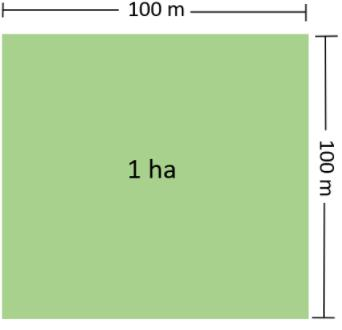# Test: Motion And Measurement Of Distances- 1

## 10 Questions MCQ Test General Science(Prelims) by IRS Divey Sethi | Test: Motion And Measurement Of Distances- 1

Description
This mock test of Test: Motion And Measurement Of Distances- 1 for UPSC helps you for every UPSC entrance exam. This contains 10 Multiple Choice Questions for UPSC Test: Motion And Measurement Of Distances- 1 (mcq) to study with solutions a complete question bank. The solved questions answers in this Test: Motion And Measurement Of Distances- 1 quiz give you a good mix of easy questions and tough questions. UPSC students definitely take this Test: Motion And Measurement Of Distances- 1 exercise for a better result in the exam. You can find other Test: Motion And Measurement Of Distances- 1 extra questions, long questions & short questions for UPSC on EduRev as well by searching above.
QUESTION: 1

### The movement of earth around the sun is an example of

Solution:

► Oscillatory motion takes place when the restoring force acting on the body is directly proportional to its displacement from its equilibrium position. In practice, oscillatory motion eventually comes to rest due to damping or frictional forces.

► Non-periodic motion : The motion which does not repeat itself after regular interval of time is called non-periodic motion. Examples : A footballer running on a field, application of brakes in a moving vehicle , a ball rolling the ground gradually slows down finally stops, motion of tides in the sea, etc.

A type of motion in which all parts of the body move the same distance in a given time is known as the translatory motion. Translatory motion can be of two types: rectilinear and curvilinear.

The movements of earth around the sun are an example of periodic motion as it is completed in fixed interval of time.

QUESTION: 2

### The standard unit of length in SI system is

Solution:

The standard unit of length in SI system is metre.

QUESTION: 3

### What device should a tailor use to measure the length of cloth?

Solution:

Measuring tape is used by a tailor to measure the length of cloth.

QUESTION: 4

One cm is equal to

Solution:

One centimetre (cm) is equal to:

► 10 mm
► 10-2m
► 10-5km

QUESTION: 5

The distance between Delhi and Kanpur is usually expressed in

Solution:

The distance between Delhi and Kanpur is usually expressed in kilometre.

QUESTION: 6

Which of the following does not represent a time-interval?

Solution:

Time of sunrise is a point of time while a day, a minute and a second represent a period of time or time interval.

QUESTION: 7

One kilometer is equal to

Solution:

One kilometre is equal to 1000 meter.

QUESTION: 8

Hectare is the unit of

Solution:

Hectare is the unit of area which is equal to 10000 meter square.QUESTION: 9

Which one is not a/an ancient unit of measurement?

Solution:

Girah, Cubit and Angul are ancient unit of measurement while meter is SI unit of measurement.

QUESTION: 10

Change in position of a body with time is called

Solution:

Change in position of a body with time is called motion.